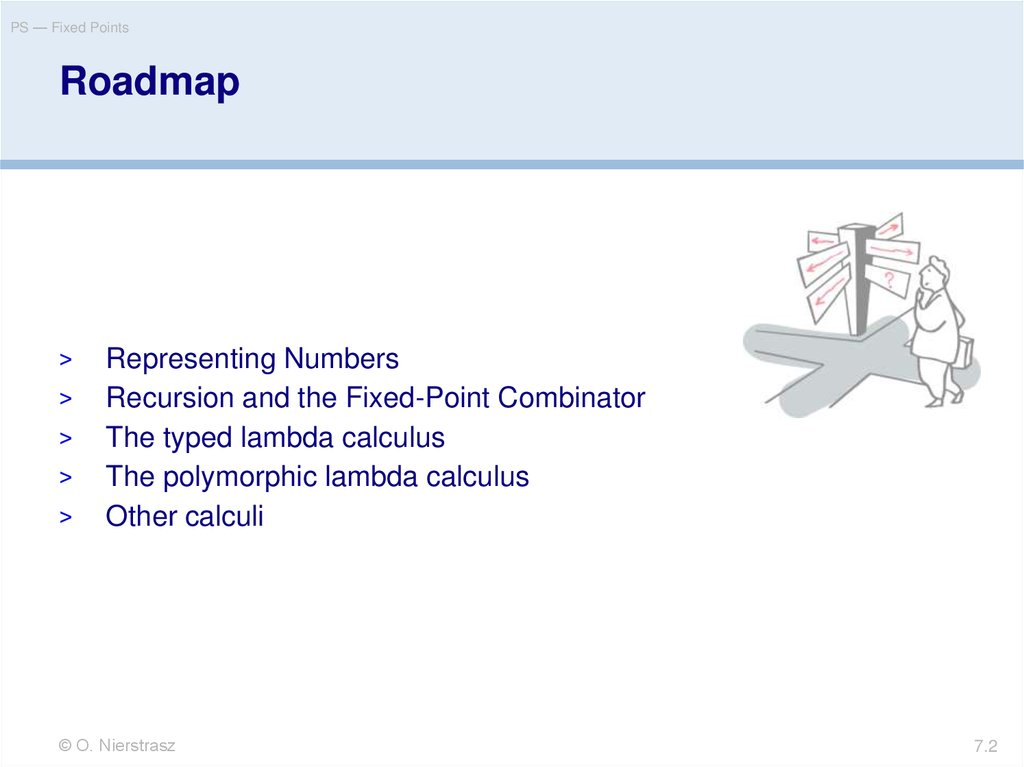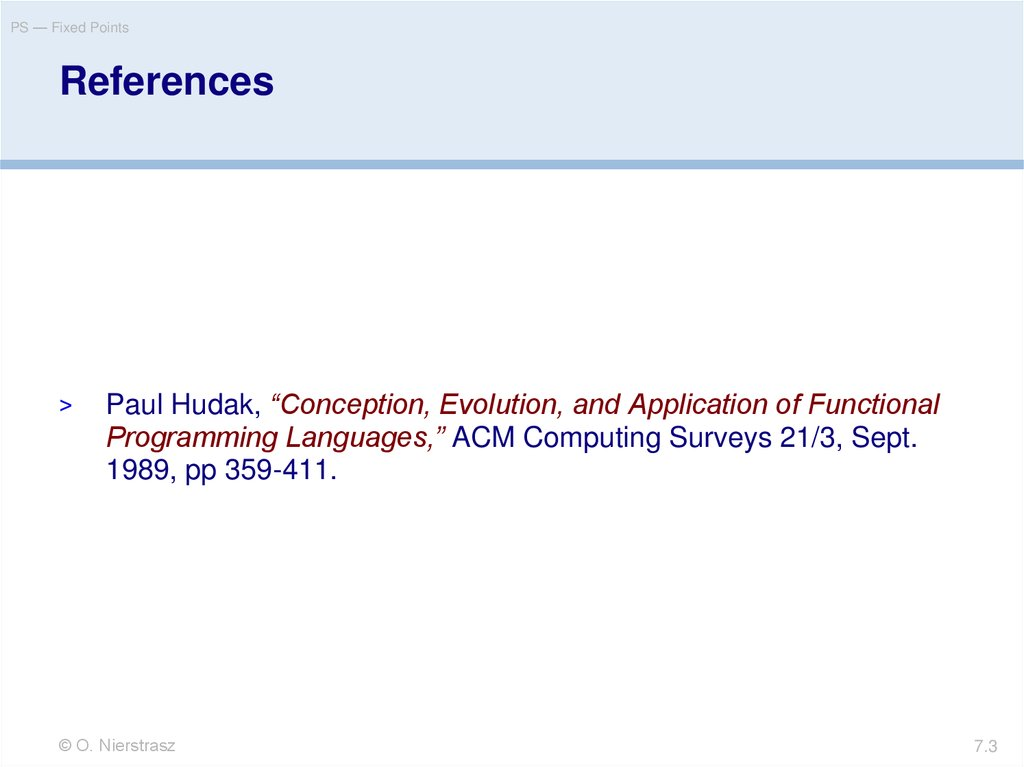# Fixed Points

## 1. 7. Fixed Points

PS — Fixed Points
>
>
>
>
>
Representing Numbers
Recursion and the Fixed-Point Combinator
The typed lambda calculus
The polymorphic lambda calculus
Other calculi
7.2

## 3. References

PS — Fixed Points
References
>
Paul Hudak, “Conception, Evolution, and Application of Functional
Programming Languages,” ACM Computing Surveys 21/3, Sept.
1989, pp 359-411.
7.3

PS — Fixed Points
>
>
>
>
>
Representing Numbers
Recursion and the Fixed-Point Combinator
The typed lambda calculus
The polymorphic lambda calculus
Other calculi
7.4

## 5. Recall these encodings …

PS — Fixed Points
Recall these encodings …
True
False
pair
(x, y)
first
second
xy.x
xy.y
( x y z . z x y)
pair x y
( p . p True )
( p . p False )
7.5

## 6. Representing Numbers

PS — Fixed Points
Representing Numbers
There is a “standard encoding” of natural numbers into the
lambda calculus:
Define:
0 ( x . x )
succ ( n . (False, n) )
then:
1 succ 0
(False, 0)
2 succ 1
(False, 1)
3 succ 2
(False, 2)
4 succ 3
(False, 3)
7.6

## 7. Working with numbers

PS — Fixed Points
Working with numbers
We can define simple functions to work with our numbers.
Consider:
iszero
first
pred
second
iszero 1
=
first (False, 0)
False
iszero 0
=
( p . p True ) ( x . x )
True
pred 1
=
second (False, 0)
0
then:
What happens when we apply pred 0? What does this mean?
7.7

PS — Fixed Points
>
>
>
>
>
Representing Numbers
Recursion and the Fixed-Point Combinator
The typed lambda calculus
The polymorphic lambda calculus
Other calculi
7.8

## 9. Recursion

PS — Fixed Points
Recursion
Suppose we want to define arithmetic operations on our lambdaencoded numbers.
plus n m
| n == 0
= m
| otherwise = plus (n-1) (m+1)
so we might try to “define”:
plus n m . iszero n m ( plus ( pred n ) ( succ m ) )
Unfortunately this is not a definition, since we are trying to use plus
before it is defined. I.e, plus is free in the “definition”!
7.9

## 10. Recursive functions as fixed points

PS — Fixed Points
Recursive functions as fixed points
We can obtain a closed expression by abstracting over plus:
rplus plus n m . iszero n
m
( plus ( pred n ) ( succ m ) )
rplus takes as its argument the actual plus function to use and returns
as its result a definition of that function in terms of itself. In other words,
if fplus is the function we want, then:
rplus fplus fplus
I.e., we are searching for a fixed point of rplus ...
7.10

## 11. Fixed Points

PS — Fixed Points
Fixed Points
A fixed point of a function f is a value p such that f p = p.
Examples:
fact 1 = 1
fact 2 = 2
fib 0 = 0
fib 1 = 1
Fixed points are not always “well-behaved”:
succ n = n + 1
What is a fixed point of succ?
7.11

## 12. Fixed Point Theorem

PS — Fixed Points
Fixed Point Theorem
Theorem:
Every lambda expression e has a fixed point p such that (e p) p.
Proof:
Let:
Y
Now consider:
p Ye
=
f . ( x . f (x x)) ( x . f (x x))
( x. e (x x)) ( x . e (x x))
e (( x . e (x x)) ( x . e (x x)))
ep
So, the “magical Y combinator” can always be used to find a
fixed point of an arbitrary lambda expression.
e: Y e e (Y e)
7.12

## 13. How does Y work?

PS — Fixed Points
How does Y work?
Recall the non-terminating expression
= ( x . x x) ( x . x x)
loops endlessly without doing any productive work.
Note that (x x) represents the body of the “loop”.
We simply define Y to take an extra parameter f, and put it into the loop,
passing it the body as an argument:
Y f . ( x . f (x x)) ( x . f (x x))
So Y just inserts some productive work into the body of
7.13

## 14. Using the Y Combinator

PS — Fixed Points
Using the Y Combinator
Consider:
f
x. True
Yf
=
f (Y f)
( x. True) (Y f)
True
by FP theorem
Y succ
succ (Y succ)
(False, (Y succ))
by FP theorem
then:
Consider:
What are succ and pred of (False, (Y succ))? What does this
represent?
7.14

## 15. Recursive Functions are Fixed Points

PS — Fixed Points
Recursive Functions are Fixed Points
We seek a fixed point of:
rplus plus n m . iszero n m ( plus ( pred n ) ( succ m ) )
By the Fixed Point Theorem, we simply take:
plus Y rplus
Since this guarantees that:
rplus plus plus
as desired!
7.15

## 16. Unfolding Recursive Lambda Expressions

PS — Fixed Points
Unfolding Recursive Lambda
Expressions
plus 1 1
=
(Y rplus) 1 1
rplus plus 1 1
(NB: fp theorem)
iszero 1 1 (plus (pred 1) (succ 1) )
False 1 (plus (pred 1) (succ 1) )
plus (pred 1) (succ 1)
rplus plus (pred 1) (succ 1)
iszero (pred 1) (succ 1)
(plus (pred (pred 1) ) (succ (succ 1) ) )
iszero 0 (succ 1) (...)
True (succ 1) (...)
succ 1
2
7.16

PS — Fixed Points
>
>
>
>
>
Representing Numbers
Recursion and the Fixed-Point Combinator
The typed lambda calculus
The polymorphic lambda calculus
Other calculi
7.17

## 18. The Typed Lambda Calculus

PS — Fixed Points
The Typed Lambda Calculus
There are many variants of the lambda calculus.
The typed lambda calculus just decorates terms with type annotations:
Syntax:
e ::= x | e1 2 1 e2 2 | ( x 2.e 1) 2 1
Operational Semantics:
x 2 . e 1
( x 2 . e1 1) e2 2
x 2. (e 1 x 2)
y 2 . [y 2/x 2] e 1
[e2 2/x 2] e1 1
e 1
y 2 not free in e 1
x 2 not free in e 1
Example:
True ( xA . ( yB . xA)B A) A (B A)
7.18

PS — Fixed Points
>
>
>
>
>
Representing Numbers
Recursion and the Fixed-Point Combinator
The typed lambda calculus
The polymorphic lambda calculus
Other calculi
7.19

## 20. The Polymorphic Lambda Calculus

PS — Fixed Points
The Polymorphic Lambda Calculus
Polymorphic functions like “map” cannot be typed in the typed lambda
calculus!
Need type variables to capture polymorphism:
reduction (ii):
( x . e1 1) e2 2 [ 2/ ] [e2 2/x ] e1 1
Example:
True
( x . ( y . x ) ) ( )
True ( ) aA bB ( y . aA ) A bB
aA
7.20

## 21. Hindley-Milner Polymorphism

PS — Fixed Points
Hindley-Milner Polymorphism
works by inferring the type annotations for a slightly restricted
subcalculus: polymorphic functions.
If:
doubleLen len len' xs ys = (len xs) + (len' ys)
then
doubleLen length length “aaa” [1,2,3]
is ok, but if
doubleLen' len xs ys = (len xs) + (len ys)
then
doubleLen' length “aaa” [1,2,3]
is a type error since the argument len cannot be assigned a unique
type!
7.21

## 22. Polymorphism and self application

PS — Fixed Points
Polymorphism and self application
Even the polymorphic lambda calculus is not powerful
enough to express certain lambda terms.
Recall that both and the Y combinator make use of “self
application”:
= ( x . x x ) ( x . x x )
What type annotation would you assign to ( x . x x)?
7.22

## 23. Built-in recursion with letrec AKA def AKA µ

PS — Fixed Points
Built-in recursion with letrec AKA def AKA µ
>
Most programming languages provide direct support for
recursively-defined functions (avoiding the need for Y)
(def f.E) e E [(def f.E) / f] e
(def plus. n m . iszero n m ( plus ( pred n ) ( succ m ))) 2 3
( n m . iszero n m ((def plus. …) ( pred n ) ( succ m ))) 2 3
(iszero 2 3 ((def plus. …) ( pred 2 ) ( succ 3 )))
((def plus. …) ( pred 2 ) ( succ 3 ))

7.23

PS — Fixed Points
>
>
>
>
>
Representing Numbers
Recursion and the Fixed-Point Combinator
The typed lambda calculus
The polymorphic lambda calculus
Other calculi
7.24

## 25. Featherweight Java

PS — Fixed Points
Featherweight Java
Used to prove that
generics could be
without breaking
the type system.
“Featherweight Java: a minimal
core calculus for Java and GJ”,
OOPSLA ’99
doi.acm.org/10.1145/320384.320395
7.25

## 26. Other Calculi

PS — Fixed Points
Other Calculi
Many calculi have been developed to study the semantics of
programming languages.
Object calculi: model inheritance and subtyping ..
— lambda calculi with records
Process calculi: model concurrency and communication
— CSP, CCS, pi calculus, CHAM, blue calculus
Distributed calculi: model location and failure
— ambients, join calculus
7.26

## 27. A quick look at the π calculus

Safety Patterns
A quick look at the π calculus
new channel
output
concurrency
input
(x)(x<z>.0 | x(y).y<x>.x(y).0) | z(v).v<v>.0
(x)(0 | z<x>.x(y).0) | z(v).v<v>.0
(x)(0 | x(y).0 | x<x>.0)
(x)(0 | 0 | 0)
en.wikipedia.org/wiki/Pi_calculus
27

## 28. What you should know!

PS — Fixed Points
What you should know!
Why isn’t it possible to express recursion directly in the
lambda calculus?
What is a fixed point? Why is it important?
How does the typed lambda calculus keep track of the
types of terms?
How does a polymorphic function differ from an ordinary
one?
7.28

## 29. Can you answer these questions?

PS — Fixed Points
How would you model negative integers in the lambda
calculus? Fractions?
Is it possible to model real numbers? Why, or why not?
Are there more fixed-point operators other than Y?
How can you be sure that unfolding a recursive
expression will terminate?
Would a process calculus be Church-Rosser?
7.29

ST — Introduction
You are free:
to Share — to copy, distribute and transmit the work
to Remix — to adapt the work
Under the following conditions:
Attribution. You must attribute the work in the manner specified by the author or licensor
(but not in any way that suggests that they endorse you or your use of the work).
Share Alike. If you alter, transform, or build upon this work, you may distribute the
resulting work only under the same, similar or a compatible license.
For any reuse or distribution, you must make clear to others the license terms of this work. The
best way to do this is with a link to this web page.
Any of the above conditions can be waived if you get permission from the copyright holder.
Nothing in this license impairs or restricts the author's moral rights.